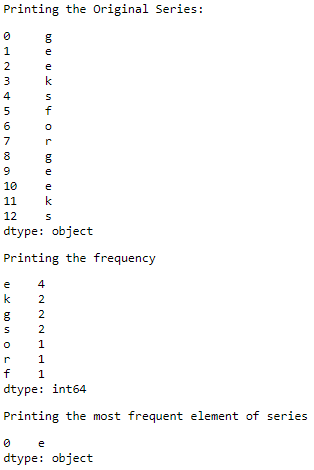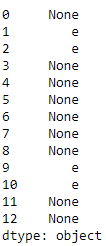# How to display most frequent value in a Pandas series?

• Last Updated : 18 Aug, 2020

In this article, our basic task is to print the most frequent value in a series. We can find the number of occurrences of elements using the value_counts() method. From that the most frequent element can be accessed by using the mode() method.

Example 1 :

 `# importing the module``import` `pandas as pd``  ` `# creating the series``series ``=` `pd.Series([``'g'``, ``'e'``, ``'e'``, ``'k'``, ``'s'``, ``                    ``'f'``, ``'o'``, ``'r'``, ``                    ``'g'``, ``'e'``, ``'e'``, ``'k'``, ``'s'``])``print``(``"Printing the Original Series:"``)``display(series)`` ` `# counting the frequency of each element``freq ``=` `series.value_counts()``print``(``"Printing the frequency"``)``display(freq)`` ` `# printing the most frequent element``print``(``"Printing the most frequent element of series"``)``display(series.mode());`

Output :Example 2 : Replacing the every element except the most frequent element with None.

 `# importing the module``import` `pandas as pd``  ` `# creating the series``series ``=` `pd.Series([``'g'``, ``'e'``, ``'e'``, ``'k'``, ``'s'``, ``                    ``'f'``, ``'o'``, ``'r'``, ``                    ``'g'``, ``'e'``, ``'e'``, ``'k'``, ``'s'``])`` ` `# counting the frequency of each element``freq ``=` `series.value_counts()`` ` `# replacing everything else as Other``series[~series.isin(freq .index[:``1``])] ``=` `None``print``(series)`

Output :My Personal Notes arrow_drop_up